Chapter 6.FOM, Problem 1P### Algebra and Trigonometry (MindTap ...

4th Edition
James Stewart + 2 others
ISBN: 9781305071742

#### Solutions

Chapter
Section### Algebra and Trigonometry (MindTap ...

4th Edition
James Stewart + 2 others
ISBN: 9781305071742
Textbook Problem
19 views

# 1-4 Modeling Periodic Data A set of data is given.(a) Make a scatter plot of the data.(b) Find a cosine function of the form y = a cos ⁡ ω t - c + b that models the data, as in Example 1.(c) Graph the function you found in part (b) together with the scatter plot. How well does the curve fit the data?(d) Use a graphing calculator to find the sine function that best fits the data, as in Example 2.(e) Compare the functions you found in parts (b) and (d). [Use the reduction formula sin ⁡ u = cos ⁡ ( u - π / 2 ) . ] t y 0 2.1 2 1.1 4 - 0.8 6 - 2.1 8 - 1.3 10 0.6 12 1.9 14 1.5

To determine

(a)

To find:

A scatter plot of the data.

Solution: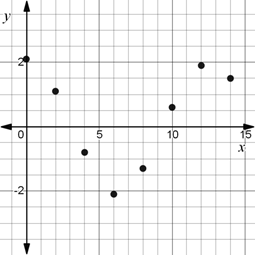Explanation

Calculation:

The scatter plot for the given data is given by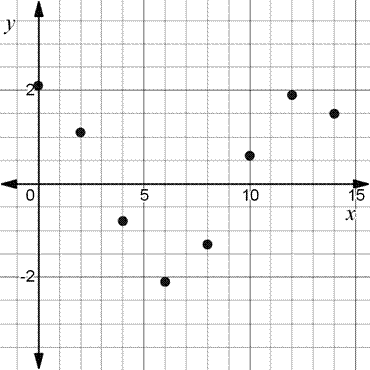Final statement: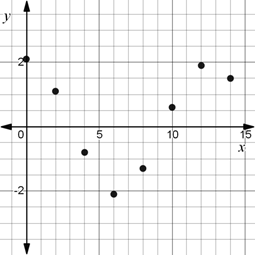To determine

(b)

To find:

A cosine function of the form y=acosωt-c+b that models the data.

Solution:

y=2.1cos0.52t.

Explanation

Calculation:

Here, the maximum value is 2.1 and the minimum value is -2.1.

The maximum value occurs at time 0 and the minimum value occurs at time 6.

The vertical shift b is the average of the maximum and minimum values.

b= vertical shift

b=12×( maximum value + minimum value)

b=122.1+-2.1

b=122.1-2.1

b=0.

The amplitude a is half of the difference between the maximum and minimum values.

a= amplitude

a=12×( maximum value - minimum value)

a=122.1--2.1

a=122.1+2.1

a=124.2

a=2.1.

The time between consecutive maximum and minimum values is half of one period.

That is, 2πω= period.

2πω=2×( time of maximum value - time of minimum value)

2πω=20-6

2πω=-12

ω=2π-12

ω=-π6.

Since the maximum value of the data occurs at t=0, it represents a cosine curve.

There is no horizontal shift.

c=0.

Apply the values in the function y=acosωt-c+b.

y=2.1cos-π6t-0+0

y=2.1cos-π6t

Since cosine is an even function, y=2.1cosπ6t.

y=2.1cos0.52t.

Final statement:

y=2.1cos0.52t.

To determine

(c)

To find:

The graph of the function from part (b).

Solution: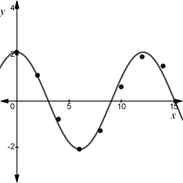Explanation

Calculation:

The graph of the function y=2.1cos0.52t is given by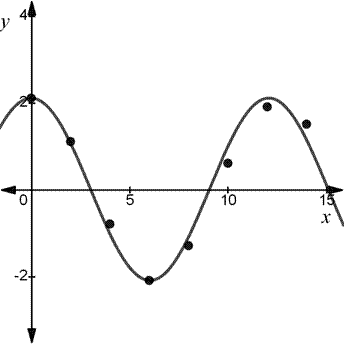Final statement: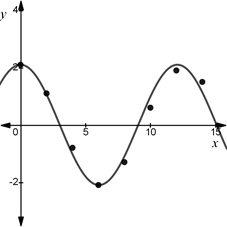To determine

(d)

To find:

The sine function that fits the data.

Solution:

y=2.05sin0.50t+1.55-0.01.

Explanation

Calculation:

Using the given data and the SinReg command on the T1-83 calculator, we get a function of the form y=asinbt+c+d.

Here, a=2.0487.2.05

b=0.5030790.50

c=1.551851.55

d=-0.008961-0.01

Apply the values in the function y=asinbt+c+d.

y=2.05sin0.50t+1.55-0.01.

Final statement:

y=2.05sin0.50t+1.55-0.01.

To determine

(e)

To find:

The comparison between the functions from part (b) and (d).

Solution:

y=2.05cos0.50t-0.02-0.01.

It is same as (b), corrected to one decimal.

Explanation

Calculation:

From part (d), y=2.05sin0.50t+1.55-0.01.

Apply the reduction formula sinx=cosx-π2.

So, y=2.05cos0.50t+1.55-π2-0.01

y=2.05cos0.50t+1.55-1.57-0.01

y=2.05cos0.50t-0.02-0.01.

It is same as (b), corrected to one decimal.

Final statement:

y=2.05cos0.50t-0.02-0.01.

It is same as (b), corrected to one decimal.

#### The Solution to Your Study Problems

Bartleby provides explanations to thousands of textbook problems written by our experts, many with advanced degrees!

Get Started